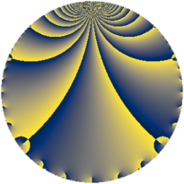# Properties

 Label 2304.2.d.bLevel $2304$ Weight $2$ Character orbit 2304.d Analytic conductor $18.398$ Analytic rank $1$ Dimension $2$ CM no Inner twists $2$

# Related objects

## Newspace parameters

 Level: $$N$$ $$=$$ $$2304 = 2^{8} \cdot 3^{2}$$ Weight: $$k$$ $$=$$ $$2$$ Character orbit: $$[\chi]$$ $$=$$ 2304.d (of order $$2$$, degree $$1$$, not minimal)

## Newform invariants

 Self dual: no Analytic conductor: $$18.3975326257$$ Analytic rank: $$1$$ Dimension: $$2$$ Coefficient field: $$\Q(\sqrt{-1})$$ Defining polynomial: $$x^{2} + 1$$ Coefficient ring: $$\Z[a_1, \ldots, a_{5}]$$ Coefficient ring index: $$2$$ Twist minimal: no (minimal twist has level 128) Sato-Tate group: $\mathrm{SU}(2)[C_{2}]$

## $q$-expansion

Coefficients of the $$q$$-expansion are expressed in terms of $$i = \sqrt{-1}$$. We also show the integral $$q$$-expansion of the trace form.

 $$f(q)$$ $$=$$ $$q + 2 i q^{5} -4 q^{7} +O(q^{10})$$ $$q + 2 i q^{5} -4 q^{7} + 2 i q^{11} + 2 i q^{13} + 2 q^{17} -2 i q^{19} -4 q^{23} + q^{25} + 6 i q^{29} -8 i q^{35} -10 i q^{37} -6 q^{41} + 6 i q^{43} -8 q^{47} + 9 q^{49} -6 i q^{53} -4 q^{55} -14 i q^{59} + 2 i q^{61} -4 q^{65} -10 i q^{67} -12 q^{71} -14 q^{73} -8 i q^{77} + 8 q^{79} -6 i q^{83} + 4 i q^{85} -2 q^{89} -8 i q^{91} + 4 q^{95} -2 q^{97} +O(q^{100})$$ $$\operatorname{Tr}(f)(q)$$ $$=$$ $$2q - 8q^{7} + O(q^{10})$$ $$2q - 8q^{7} + 4q^{17} - 8q^{23} + 2q^{25} - 12q^{41} - 16q^{47} + 18q^{49} - 8q^{55} - 8q^{65} - 24q^{71} - 28q^{73} + 16q^{79} - 4q^{89} + 8q^{95} - 4q^{97} + O(q^{100})$$

## Character values

We give the values of $$\chi$$ on generators for $$\left(\mathbb{Z}/2304\mathbb{Z}\right)^\times$$.

 $$n$$ $$1279$$ $$1793$$ $$2053$$ $$\chi(n)$$ $$1$$ $$1$$ $$-1$$

## Embeddings

For each embedding $$\iota_m$$ of the coefficient field, the values $$\iota_m(a_n)$$ are shown below.

For more information on an embedded modular form you can click on its label.

Label $$\iota_m(\nu)$$ $$a_{2}$$ $$a_{3}$$ $$a_{4}$$ $$a_{5}$$ $$a_{6}$$ $$a_{7}$$ $$a_{8}$$ $$a_{9}$$ $$a_{10}$$
1153.1
 − 1.00000i 1.00000i
0 0 0 2.00000i 0 −4.00000 0 0 0
1153.2 0 0 0 2.00000i 0 −4.00000 0 0 0
 $$n$$: e.g. 2-40 or 990-1000 Significant digits: Format: Complex embeddings Normalized embeddings Satake parameters Satake angles

## Inner twists

Char Parity Ord Mult Type
1.a even 1 1 trivial
8.b even 2 1 inner

## Twists

By twisting character orbit
Char Parity Ord Mult Type Twist Min Dim
1.a even 1 1 trivial 2304.2.d.b 2
3.b odd 2 1 256.2.b.a 2
4.b odd 2 1 2304.2.d.r 2
8.b even 2 1 inner 2304.2.d.b 2
8.d odd 2 1 2304.2.d.r 2
12.b even 2 1 256.2.b.c 2
16.e even 4 1 1152.2.a.h 1
16.e even 4 1 1152.2.a.r 1
16.f odd 4 1 1152.2.a.c 1
16.f odd 4 1 1152.2.a.m 1
24.f even 2 1 256.2.b.c 2
24.h odd 2 1 256.2.b.a 2
48.i odd 4 1 128.2.a.b yes 1
48.i odd 4 1 128.2.a.c yes 1
48.k even 4 1 128.2.a.a 1
48.k even 4 1 128.2.a.d yes 1
96.o even 8 4 1024.2.e.i 4
96.p odd 8 4 1024.2.e.m 4
240.t even 4 1 3200.2.a.h 1
240.t even 4 1 3200.2.a.x 1
240.z odd 4 1 3200.2.c.e 2
240.z odd 4 1 3200.2.c.l 2
240.bb even 4 1 3200.2.c.f 2
240.bb even 4 1 3200.2.c.k 2
240.bd odd 4 1 3200.2.c.e 2
240.bd odd 4 1 3200.2.c.l 2
240.bf even 4 1 3200.2.c.f 2
240.bf even 4 1 3200.2.c.k 2
240.bm odd 4 1 3200.2.a.e 1
240.bm odd 4 1 3200.2.a.u 1
336.v odd 4 1 6272.2.a.a 1
336.v odd 4 1 6272.2.a.h 1
336.y even 4 1 6272.2.a.b 1
336.y even 4 1 6272.2.a.g 1

By twisted newform orbit
Twist Min Dim Char Parity Ord Mult Type
128.2.a.a 1 48.k even 4 1
128.2.a.b yes 1 48.i odd 4 1
128.2.a.c yes 1 48.i odd 4 1
128.2.a.d yes 1 48.k even 4 1
256.2.b.a 2 3.b odd 2 1
256.2.b.a 2 24.h odd 2 1
256.2.b.c 2 12.b even 2 1
256.2.b.c 2 24.f even 2 1
1024.2.e.i 4 96.o even 8 4
1024.2.e.m 4 96.p odd 8 4
1152.2.a.c 1 16.f odd 4 1
1152.2.a.h 1 16.e even 4 1
1152.2.a.m 1 16.f odd 4 1
1152.2.a.r 1 16.e even 4 1
2304.2.d.b 2 1.a even 1 1 trivial
2304.2.d.b 2 8.b even 2 1 inner
2304.2.d.r 2 4.b odd 2 1
2304.2.d.r 2 8.d odd 2 1
3200.2.a.e 1 240.bm odd 4 1
3200.2.a.h 1 240.t even 4 1
3200.2.a.u 1 240.bm odd 4 1
3200.2.a.x 1 240.t even 4 1
3200.2.c.e 2 240.z odd 4 1
3200.2.c.e 2 240.bd odd 4 1
3200.2.c.f 2 240.bb even 4 1
3200.2.c.f 2 240.bf even 4 1
3200.2.c.k 2 240.bb even 4 1
3200.2.c.k 2 240.bf even 4 1
3200.2.c.l 2 240.z odd 4 1
3200.2.c.l 2 240.bd odd 4 1
6272.2.a.a 1 336.v odd 4 1
6272.2.a.b 1 336.y even 4 1
6272.2.a.g 1 336.y even 4 1
6272.2.a.h 1 336.v odd 4 1

## Hecke kernels

This newform subspace can be constructed as the intersection of the kernels of the following linear operators acting on $$S_{2}^{\mathrm{new}}(2304, [\chi])$$:

 $$T_{5}^{2} + 4$$ $$T_{7} + 4$$ $$T_{11}^{2} + 4$$ $$T_{17} - 2$$ $$T_{23} + 4$$

## Hecke characteristic polynomials

$p$ $F_p(T)$
$2$ $$T^{2}$$
$3$ $$T^{2}$$
$5$ $$4 + T^{2}$$
$7$ $$( 4 + T )^{2}$$
$11$ $$4 + T^{2}$$
$13$ $$4 + T^{2}$$
$17$ $$( -2 + T )^{2}$$
$19$ $$4 + T^{2}$$
$23$ $$( 4 + T )^{2}$$
$29$ $$36 + T^{2}$$
$31$ $$T^{2}$$
$37$ $$100 + T^{2}$$
$41$ $$( 6 + T )^{2}$$
$43$ $$36 + T^{2}$$
$47$ $$( 8 + T )^{2}$$
$53$ $$36 + T^{2}$$
$59$ $$196 + T^{2}$$
$61$ $$4 + T^{2}$$
$67$ $$100 + T^{2}$$
$71$ $$( 12 + T )^{2}$$
$73$ $$( 14 + T )^{2}$$
$79$ $$( -8 + T )^{2}$$
$83$ $$36 + T^{2}$$
$89$ $$( 2 + T )^{2}$$
$97$ $$( 2 + T )^{2}$$